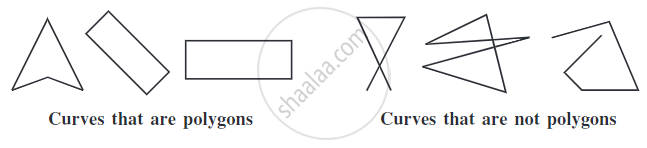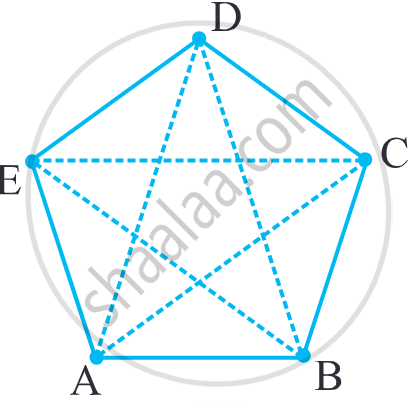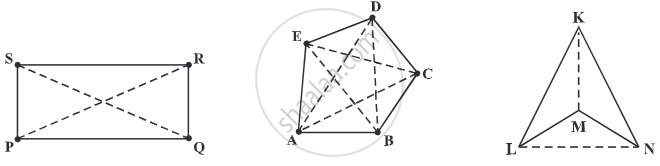# Concept of Polygons - Side, Vertex, Adjacent Sides, Adjacent Vertices and Diagonal

## Definition

Polygon: Polygon refers to a closed 2D shape which is made up of a finite number of the line segments.

Sides: The line segments forming a shape are called its sides.

Vertex: The meeting point of a pair of sides is called its vertex.

Adjacent sides: Any two sides with a common endpoint are called the adjacent sides.

Adjacent vertices: The endpoints of the same side of a polygon are called the adjacent vertices.

Diagonal: A diagonal is a line segment connecting two non-consecutive vertices of a polygon.

## Notes

### Polygons:

• A polygon is a simple closed curve made up of line segments.

• Polygon comes from Greek. Poly- means "many" and -gon means "angle".

• Polygon refers to a closed 2D shape which is made up of a finite number of the line segments.

•• Here,• The line segments forming a polygon are called its sides.
Sides are bar"AB", bar"BC", bar"CD", bar"DE" and bar"EA".

• The meeting point of a pair of sides is called its vertex.
Sides bar "AE" and bar "ED" meet at E,
so E is a vertex of the polygon ABCDE.

• Any two sides with a common endpoint are called the adjacent sides of the polygon.
Sides bar "AB" and bar "BC" are adjacent sides.

• The endpoints of the same side of a polygon are called the adjacent vertices.
Vertices E and D are adjacent, whereas vertices A and D are not adjacent vertices.

• A diagonal is a line segment connecting two non-consecutive vertices of a polygon.
bar"AC", bar"AD", bar"BD", bar"BE" and bar"CE" are diagonals.If you would like to contribute notes or other learning material, please submit them using the button below.

### Shaalaa.com

What are Polygons? [00:06:22]
S
0%